Home | | Maths 12th Std | EulerŌĆÖs Form of the complex number

# EulerŌĆÖs Form of the complex number

When performing multiplication or finding powers or roots of complex numbers, Euler form can also be used.

EulerŌĆÖs Form of the complex number

The following identity is known as EulerŌĆÖs formula

ei╬Ė  = cos╬Ė + i sin╬Ė

Euler formula gives the polar form z = r ei╬Ė

Note

When performing multiplication or finding powers or roots of complex numbers, Euler form can also be used.

Example 2.22

Find the modulus and principal argument of the following complex numbers.

(i) ŌłÜ3 + i

(ii) -ŌłÜ3 + i

(iii) - ŌłÜ3 - i

(iv) ŌłÜ3 - i

Solution

(i) ŌłÜ3 + i

Modulus =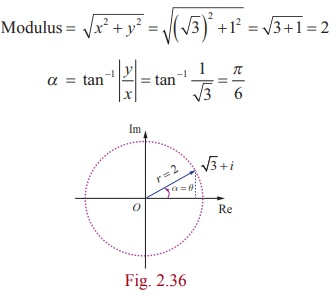Since the complex number has the principal value ŌłÜ3 + i lies in the first quadrant, has the principal value

╬Ė = ╬▒ = ŽĆ/6.

Therefore, the modulus and principal argument of ŌłÜ3 + i are 2 and ŽĆ/6 respectively.

(ii) -ŌłÜ3 + i

Modulus = 2 andSince the complex number  -ŌłÜ3 + i lies in the second quadrant has the principal valueTherefore the modulus and principal argument of (ii) -ŌłÜ3 + i are 2 and 5ŽĆ/6 respectively.

(iii) - ŌłÜ3 - i

r = 2 and ╬▒ = ŽĆ /6 .

Since the complex number - ŌłÜ3 - i lies in the third quadrant, has the principal value,Therefore, the modulus and principal argument of - ŌłÜ3 - i are 2 and -5ŽĆ/6 respectively.

(iv) ŌłÜ3 - i

r = 2 and ╬▒ = ŽĆ/6

Since the complex number lies in the fourth quadrant, has the principal value,

╬Ė = -╬▒ = -ŽĆ/6Therefore, the modulus and principal argument of

ŌłÜ3 - i  are 2 and - ŽĆ/6 .

In all the four cases, modulus are equal, but the arguments are depending on the quadrant in which the complex number lies.

Example 2.23

Represent the complex number (i) -1- i (ii) 1+ iŌłÜ3 in polar form.

Solution

(i) Let -1- i = r(cos╬Ė + isin╬Ė )

We have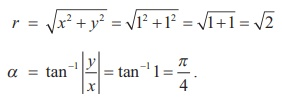Since the complex number -1- i lies in the third quadrant, it has the principal value,Note

Depending upon the various values of k , we get various alternative polar forms.

(ii) 1+ iŌłÜ3Therefore, the polar form of 1+ iŌłÜ3 can be written as### Example 2.24

Find the principal argument Arg z , when z = -2 / [ 1+iŌłÜ3 ].

### SolutionThis implies that one of the values of arg z is 2ŽĆ/3 .

Since 2ŽĆ/3 lies between -ŽĆ and ŽĆ, the principal argument Arg z is 2 ŽĆ/3.

## Properties of polar form

### Property 1

If z = r (cos╬Ė + i sin╬Ė ), then z-1 =1/r  (cos╬Ė - i sin╬Ė ) .

### Proof### Property 2

If z1  = r1 (cos╬Ė1  + i sin╬Ė1 ) and  z2  = r2 (cos╬Ė2  + i sin╬Ė2 ) ,

then   z1 z2  = r1r2 (cos (╬Ė1  + ╬Ė2 ) + i sin (╬Ė1  + ╬Ė2 )) .

### Proof

z = r (cos╬Ė1 + i sin╬Ė1) and

= r (cos╬Ė2  + i sin╬Ė2)ŌćÆ z1 z2   =  r1 (cos╬Ė1  + i sin╬Ė1 ) r2 (cos╬Ė2  + i sin╬Ė2 )

r1r2 ((cos╬Ė1 cos╬Ė2  - sin╬Ė1 sin╬Ė2 ) + i (sin╬Ė1 cos╬Ė2  + sin╬Ė2  cos╬Ė1 ))

z1 z2   =  r1r2 (cos (╬Ė1  + ╬Ė2 ) + i sin (╬Ė1  + ╬Ė2 )) .

### Note

arg ( z1 z2 ) = ╬Ė1 + ╬Ė2 = arg ( z1 ) + arg ( z2 ) .

### Property 3Proof

Using the polar form of z1 and z2, we have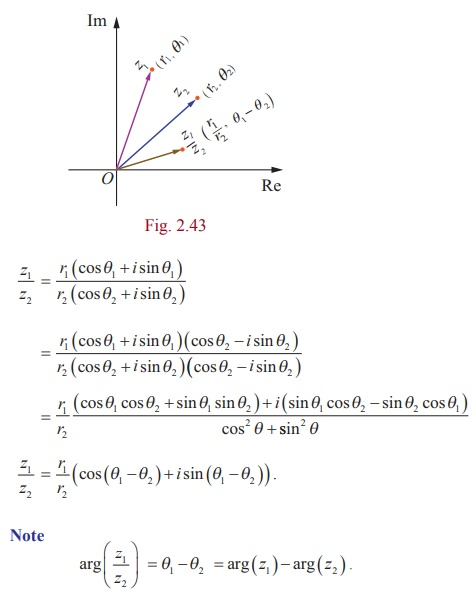Example 2.25

Find the productin rectangular from.

Solution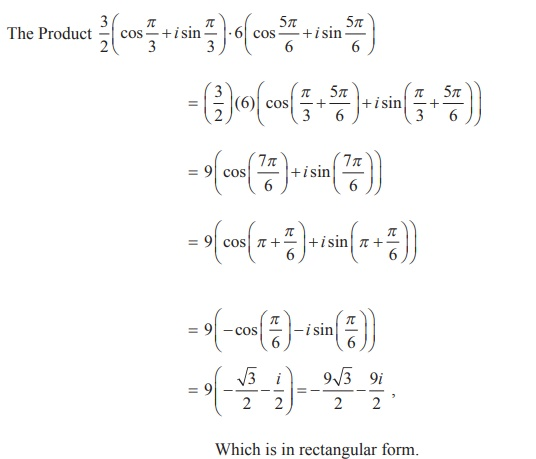Example 2.26

Find the quotientin rectangular form.

SolutionWhich is in rectangular form.

Example 2.27

If z = x + iy and, show that x2 + y2 =1.

Solution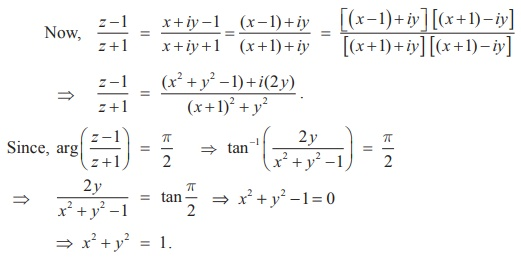ŌćÆ x2 + y2 =1

Tags : Definition, Properties, Formulas, Solved Example Problems , 12th Mathematics : UNIT 2 : Complex Numbers
Study Material, Lecturing Notes, Assignment, Reference, Wiki description explanation, brief detail
12th Mathematics : UNIT 2 : Complex Numbers : EulerŌĆÖs Form of the complex number | Definition, Properties, Formulas, Solved Example Problems